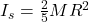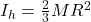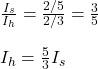Question

Two spheres have the same radius and equal masses. One is made of solid aluminum, and the other is made from a hollow shell of gold. Which one has the bigger moment of inertia about an axis through its center?

1.MichaelMetExplanation:

In order to know which sphere has the bigger moment of inertia, you use the following formulas for the moment of inertia for each solid sphere and hollow shell. Both spheres have the same radius and mass.

Solid sphere:(1)

Hollow shell:(2)

If you dive the equation (1) into the equation (2) you obtain:Then, the hollow shell has a moment of inertia that is 3/5 times the moment of inertia of the solid sphere.